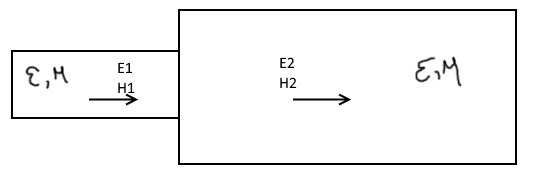# I Reflection (intrinsic and characteristic impedance effects) (1 Viewer)

### Users Who Are Viewing This Thread (Users: 0, Guests: 1)

#### iVenky

Do we have reflection when the intrinsic impedance η=E/H between two media are matched but not necessarily the characteristic impedance (assuming a transmission line)?

Basically, I have a case here shown belowI have two parts with different geometries (this may not be a transmission-line, if you want you can assume an equivalent structure for a parallel line to get a transmission-line). The material parameters μ, ε are the same between the two media but their geometry is different. Do we still have a reflection at the boundary in this case? (does the boundary condition result in a reflection?)

#### Attachments

• 7.1 KB Views: 147

#### tech99

Gold Member
Allow me to talk in terms of transmission lines, and let me assume they have resistive characteristic impedances Z1 and Z2.
LIne 2 is shown as transmitting energy to the right, so we assume it is terminated at the right hand edge with a matching resistor so there are no reflections. Alternatively it might be very long, so reflected energy is absorbed.
Line 2 it therefore presents a resistive input impedance equal to its characteristic impedance, Z2. It looks like a resistor.
Line 1 has different characteristic impedance to Z2, so it will always be mismatched and will have standing waves. There is always reflection at the boundary.
It is possible to obtain a resistive source impedance into line 1 for lengths where line 1 is resonant (quarter or half wave in length), but line 1 itself will always have standing waves, showing it is mismatched.
It is possible to insert a matching device at the junction, so that line 1 sees a ratio of E and H equal to its characteristic impedance, but that is not, I think, your question.

•iVenky

#### iVenky

Allow me to talk in terms of transmission lines, and let me assume they have resistive characteristic impedances Z1 and Z2.
LIne 2 is shown as transmitting energy to the right, so we assume it is terminated at the right hand edge with a matching resistor so there are no reflections. Alternatively it might be very long, so reflected energy is absorbed.
Line 2 it therefore presents a resistive input impedance equal to its characteristic impedance, Z2. It looks like a resistor.
Line 1 has different characteristic impedance to Z2, so it will always be mismatched and will have standing waves. There is always reflection at the boundary.
It is possible to obtain a resistive source impedance into line 1 for lengths where line 1 is resonant (quarter or half wave in length), but line 1 itself will always have standing waves, showing it is mismatched.
It is possible to insert a matching device at the junction, so that line 1 sees a ratio of E and H equal to its characteristic impedance, but that is not, I think, your question.
Hi,

Thanks for the reply. Do you mean to say that E/H ratios of two lines aren't matched if the dimensions are different? I was always thinking that the source of reflection was the mismatch of E/H ratio and the boundary condition. But, I read recently in a book that you can have same E/H ratios but different characteristic impedance, which may depend on dimension of the line, and this can result in reflections. Hence the confusion.

#### tech99

Gold Member
Hi,

Thanks for the reply. Do you mean to say that E/H ratios of two lines aren't matched if the dimensions are different?
Yes. The ratio of diameter and spacing needs to be the same (assuming the same dielectric).

•iVenky

#### iVenky

Yes. The ratio of diameter and spacing needs to be the same (assuming the same dielectric).
Thanks now that answers my question

### The Physics Forums Way

We Value Quality
• Topics based on mainstream science
• Proper English grammar and spelling
We Value Civility
• Positive and compassionate attitudes
• Patience while debating
We Value Productivity
• Disciplined to remain on-topic
• Recognition of own weaknesses
• Solo and co-op problem solving